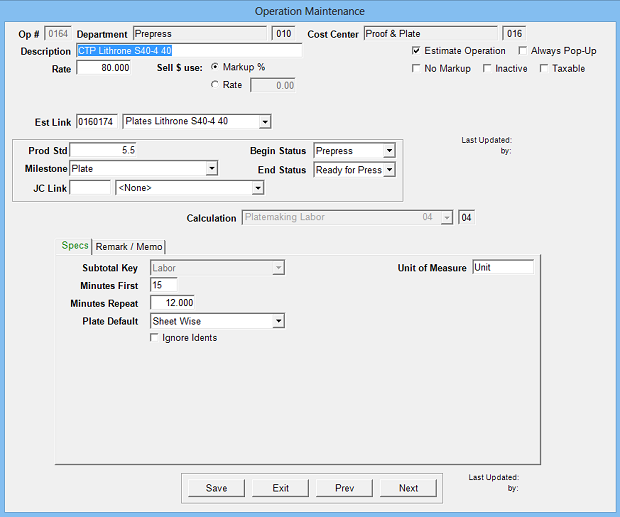Estimates the cost and time of platemaking.

Operation Setup Requirements:
1. Description
2. Rate: Cost per hour
3. Sell \$ use: Markup % (recommended) or alternate sell rate
4. Calc: 4
5. Sub Key: Labor
6. Minutes First: Minutes to make first plate
7. Minutes Repeat: Minutes for each additional plate
8. Plate Default: Sheetwise, Work & Turn, Work & Tumble, or Web OnlyEstimate Specification Input Prompts:

1. Idents
2. Plate Type
3. Plates
4. J%
5. Remarks
6. MemoCalculation:

Colors Side A + Colors Side B (divided by 2 if Work and Turn or Work and Tumble) = Total Colors

Total Colors x Idents (or override) = Total Plates

Minutes First + (Total Plates - 1) x Minutes Repeat = Standard Minutes

Standard Minutes / 60 x J% = Estimated Hours

Estimated Hours x Rate = Cost \$

Cost \$ x Markup % = Markup \$

Cost \$ + Markup \$ = Sell \$

Note: Calc 4 followed by a Calc 5 will automatically calculate plate material